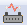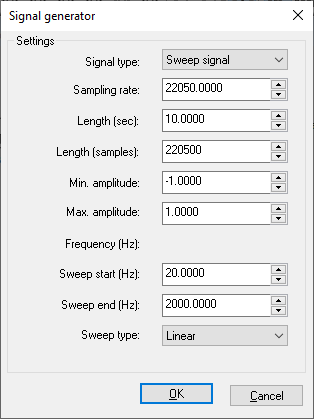If you need to generate artificial signal by using some standard mathematical function, use the “Signal tools/Signal generator” option from the menu or thetoolbar button. You can choose the type of the function (sine, step, white noise…), signal length, frequency, sample rate, min & max values... SIGVIEW will only allow input of values which make sense for the chosen signal type (for example, there is no frequency for a white noise signal) and disable all other fields.

After you set all signal parameters, click OK and a window with defined signal will be created. You can work with this signal as with any other signal or you can save it in a file for later use. If you would like to use this signal for sound output, simply select the “Play & navigate /Play signal (with sound)” option from the main menu. Choose the “Repeat forever” menu option if you would like to play the signal continuously.The following signal types are supported by the signal generator:

• Sine, Cosine: "Pure frequency" signals generated by using corresponding mathematical functions
• Step: Also known as "square wave". Changes its amplitude from minimal to maximal value according to frequency setting
• White noise: Random signal with a flat distribution of random values
• Pink noise: Also known as 1/f noise. A random signal where each octave carriers an equal amount of noise power, i.e. signal power falls as frequency raises
• Sawtooth: This signal ramps linearly from minimal to maximal value and then sharply drops to the minimal value again. Its waveform resembles the teeth on the blade of a saw
• Triangular: Similar to sawtooth signals but also falls linearly from maximal to minimal value. Each period forms a triangular form
• Constant: All signal vales are the same
• Gaussian noise: Statistical noise that has a probability density function of the normal distribution (also known as Gaussian distribution). In other words, the values that the noise can take on are Gaussian-distributed
• Exponential noise: Statistical noise that has a probability density function of the exponential distribution. In other words, the values that the noise can take on are exponentially distributed
• Sweep signal: This is a periodic (sine-like) signal which changes (sweeps) its frequency from defined start to end frequency during signal duration. There are two types of sweep signals: linear sweeps through frequency range at linear speed and exponential speeds up exponentially during sweeping so that lower frequencies have longer duration than higher frequencies.